[BACK]
 Computers, Materials & ContinuaDOI:10.32604/cmc.2022.022214Article

Partially Overlapping Channel Assignment Using Bonded and Non-Bonded Channels in IEEE 802.11n WLAN

1Graduate School of Natural Science and Technology, Okayama University, Okayama, 700-8530, Japan
2Computer Science and Engineering, Jatiya Kabi Kazi Nazrul Islam University, Mymensingh, 2224, Bangladesh
*Corresponding Author: Fatema Akhter. Email: fatema@s.okayama-u.ac.jp
Received: 31 July 2021; Accepted: 06 September 2021

Abstract: Nowadays, wireless local area network (WLAN) has become prevalent Internet access due to its low-cost gadgets, flexible coverage and hassle-free simple wireless installation. WLAN facilitates wireless Internet services to users with mobile devices like smart phones, tablets, and laptops through deployment of multiple access points (APs) in a network field. Every AP operates on a frequency band called channel. Popular wireless standard such as IEEE 802.11n has a limited number of channels where frequency spectrum of adjacent channels overlaps partially with each other. In a crowded environment, users may experience poor Internet services due to channel collision i.e., interference from surrounding APs that affects the performance of the WLAN system. Therefore, it becomes a challenge to maintain expected performance in a crowded environment. A mathematical model of throughput considering interferences from surrounding APs can play an important role to set up a WLAN system properly. While set up, assignment of channels considering interference can maximize network performance. In this paper, we investigate the signal propagation of APs under interference of partially overlapping channels for both bonded and non-bonded channels. Then, a throughput estimation model is proposed using difference of operating channels and received signal strength indicator (RSSI). Then, a channel assignment algorithm is introduced using proposed throughput estimation model. Finally, the efficiency of the proposal is verified by numerical experiments using simulator. The results show that the proposal selects the best channel combination of bonded and non-bonded channels that maximize the performance.

Keywords: Wireless local area network; partially overlapping channel; throughput estimation model; channel assignment algorithm

1  Introduction

Wireless local area network (WLAN) provides ubiquitous and flexible wireless Internet service to users at low cost. In a WLAN framework, users can move freely without losing connections while communicating through Internet. Recent advancements in wireless technology have brought an assortment of inexpensive gadgets for Internet access. Hence, WLAN has become increasingly popular today as low-cost Internet access among versatile users with handy gadgets . WLANs are commonly deployed in places like homes, schools, offices, hotels, railway stations, airports and shopping malls [3,4]. Access points (APs) are often installed in a spontaneous manner which can cause wide spread of APs in a small network field. In such a dense network environment, coverage areas of APs can overlap each other where they may use same channel of frequency signal. This could result in poor network performance due to interference among APs. Therefore, the channels of these APs ought to be set up appropriately to avoid interference so as to enhance network performance.

In IEEE 802.11 standard, WLANs operate in two unlicensed frequency spectrum bands: the 2.4 GHz Industrial, Scientific, and Medical band (ISM) and the 5 GHz Unlicensed National Information Infrastructure band (U-NII) [5,6]. The IEEE 802.11 WLAN standard characterizes a fixed number of channels for use by APs and their users. A channel is specified a bandwidth of 22 MHz width and is separated by 5 MHz from center frequency of its adjacent channel. Such channel is referred to as 20 MHz channel and conventionally knows as non-bonded channel. The signal falls within about 11 MHz of each side of the center frequency. Therefore, signal on any channel partially overlaps with several adjacent channels. Conventionally, they are referred to as partially overlapping channels (POCs). Typically, IEEE 802.11n standard supports 13 channels where only 3 channels are free from overlapping one another and referred to as orthogonal channels (OCs) [5,7]. The increasing demand of high performance resulted in channel bonding mechanism, where two adjacent 20 MHz channels are combined together to form one 40 MHz channel [8,9] known as bonded channel. Bonded channels offer higher data transmission. However, this reduces the number of channels both POCs and OCs. Fig. 1 illustrates available channels in 2.4 GHz spectrum for IEEE 802.11n standard.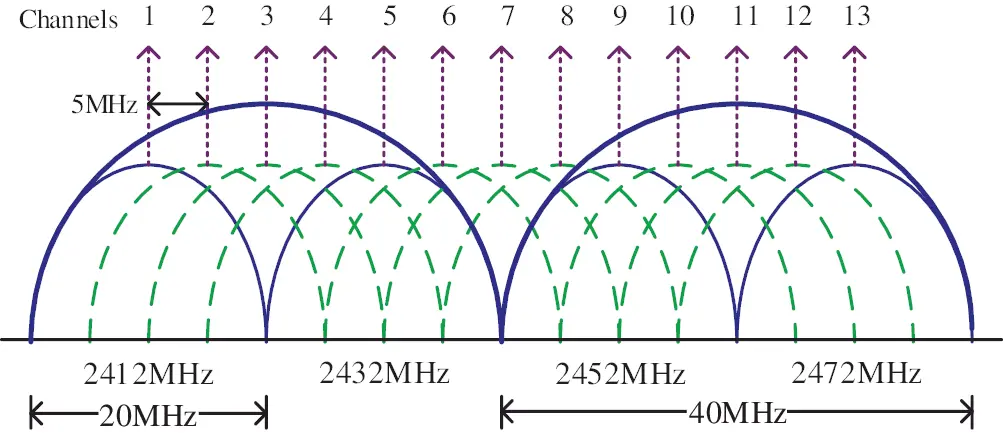Figure 1: Available channels in IEEE 802.11n for 2.4 GHz band

Several researches are conducted to derive interference models among operating APs and reduce interference by allocating OCs and POCs properly. In , authors proposed a network interference model based on signal-to-interference-plus-noise ratio (SINR). A direct relationship between maximizing system throughput and minimizing total interference is derived for POCs. Then, a channel assignment algorithm is proposed to minimize the total interference in network. The algorithm is a simple greedy one that fails often to find optimal solutions. In , authors proposed a channel allocation method using an interference model where APs are operating in point coordination function (PCF) mode. Only orthogonal channels are adopted for allocation. An interference probability function is introduced based on interference relationship among hosts. However, the authors avoided degree of interference in the proposed model. In , authors proposed an interference model based on two key features of IEEE 802.11n i.e., channel bonding and frame aggregation mechanism. A distributed channel allocation method for multi-rate 802.11n network is proposed considering estimated throughput of each client in network system. In , authors proposed similar distributed channel allocation method based on the interfering relation among clients in the network. The algorithms pay higher cost to exchange information among hosts. In [16,17], authors proposed a joint channel allocation and power control method to reduce interference problem. However, competition among APs and load distributions are not considered to eliminate interference completely.

While conventional channel assignment algorithms are designed to optimize communication performances by reducing interference only for 20 MHz channel [2,18,19], in this work, we propose an interference model for both 20 and 40 MHz channels in IEEE 802.11n WLAN system and a channel assignment algorithm under POCs using proposed interference model. The proposed interference model utilizes overlapping degree of 20 MHz channels studied in  and extends it for 40 MHz channels. In addition, a link speed estimation model using received signal strength indicator (RSSI) is developed for both 20 and 40 MHz channels. Then, we formulate a hierarchical optimization problem for channel assignment algorithm that reduces total interference among APs in a network with an aim to maximize network performance. We named the proposed algorithm POCABnB (partially overlapping channel assignment using bonded and non-bonded channels). One bit communication time of APs is considered for the communication performance metric in this work as it is crucial for many applications maintaining fairness criterion to guarantee a minimum throughput to every user in a WLAN system.

The proposed channel assignment algorithm builds a weighted inference graph from network topology where an edge represents existence of interference and weight value represents degree of interference between connected APs. An exhaustive channel assignment can ensure optimal network performance. However, such procedure has exponential time complexity. Therefore, we utilized a greedy method to derive an initial solution for channel assignment. Then, the solution is improved iteratively using simulated annealing algorithm. After assignment of channels, load on different channels may become imbalance that can affect communication performance. Therefore, we average load on channels by changing associations of some users to APs. Finally, the proposal is evaluated by simulations under different network environments. In short, the contributions of this study are listed below:

① A channel interference model using both 20 and 40 MHz channel width for IEEE 802.11n protocol.

② A channel assignment algorithm under POCs using proposed channel interference model for WLAN system.

③ Evaluation of proposed channel assignment algorithm using simulation under different network topologies.

The rest of this paper is organized as follows: the proposed interference model for IEEE 802.11n protocol, link speed estimation model for 20 and 40 MHz channel and optimization problem formulation for proposed channel assignment algorithm are introduced in Section 2. Proposed channel assignment algorithm and channel load balancing methods are described in Section 3. Experimental results to demonstrate the effectiveness of the proposed channel assignment algorithm are presented in Section 4. Finally, Section 5 concludes this paper with some future works.

2  System Model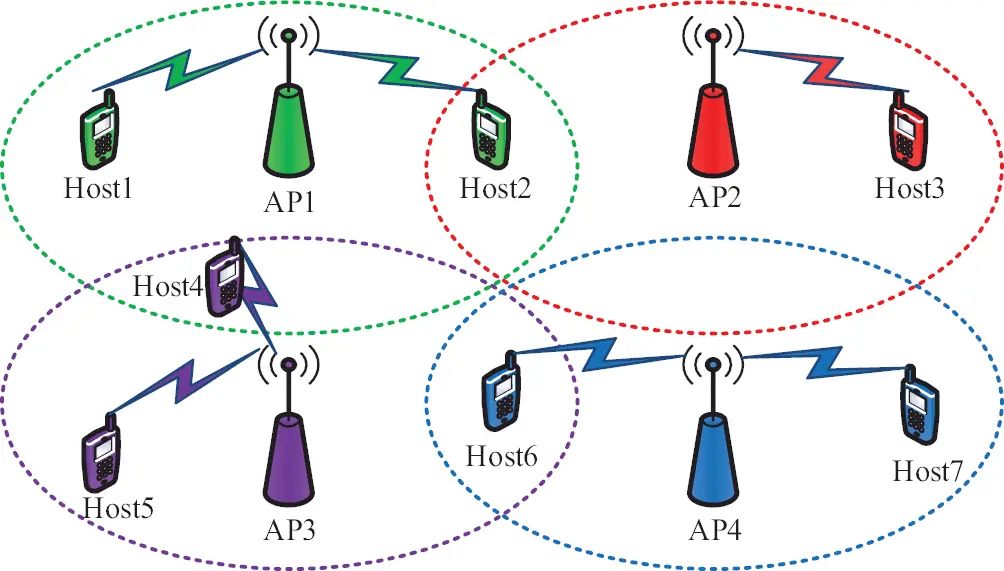Figure 2: Illustration of a WLAN system deployment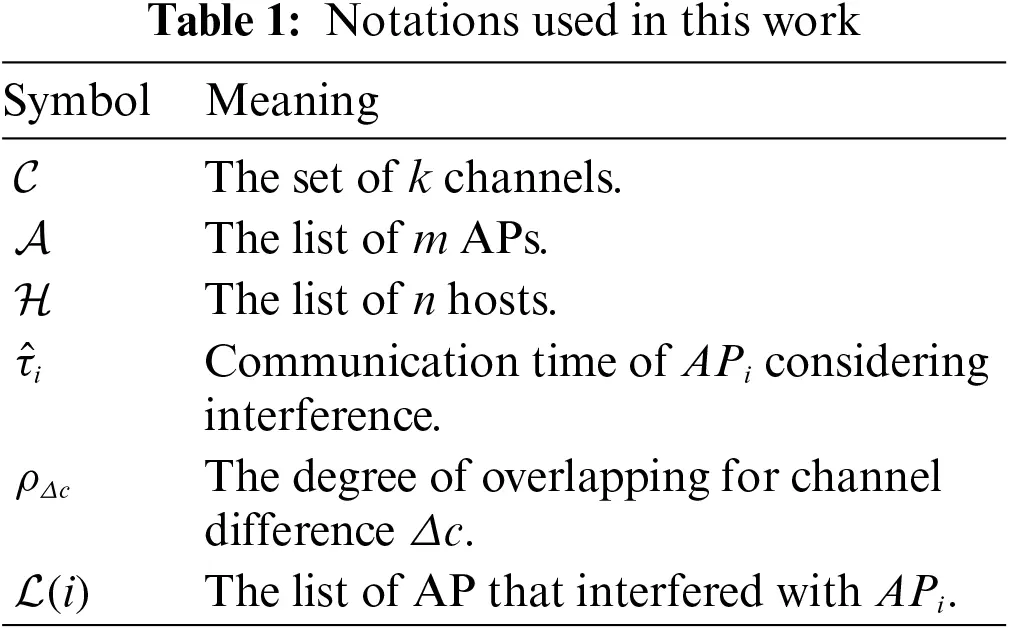2.1 Interference Model

Within the scope of this work, we focus on channel assignment algorithm using POCs in the context of both 20 and 40 MHz channels. A 40 MHz channel is a combination of two adjacent 20 MHz channels. The list of bonded channels is demonstrated in Tab. 2. The degree of interference among channels has been widely studied where only few of them reported on bonded channels. This work considers degree of interference among channels for both bonded and non-bonded channels.The estimation of the degree of interference between two channels is a difficult task. For 20 MHz channel, there has been a popular study for measurement of overlapping degree in  which is summarized in Tab. 3. Where Δc represents the difference between two channels and ρΔc does the overlapping degree for the respective Δc.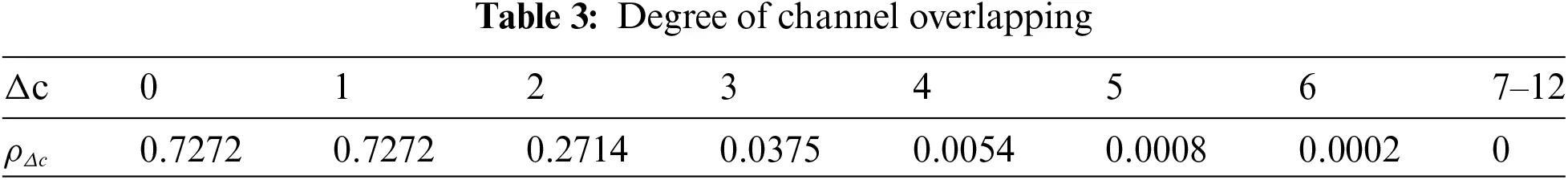In this work, we utilized this overlapping degree to derive interference model for proposed channel assignment algorithm. If APi and APj are operating on channels ci and cj respectively, the channel difference Δc=|cicj| and degree of interference ρi,j is defined as follows:

whenbothciandcjare20MHz:Δc=|cicj|,ρi,j=ρj,i=ρΔcwhenandbothciandcjare40MHz:if|cicj|4:Δc=|cicj|,ρi,j=ρj,i=1+ρΔc2else:Δc=|cicj4|,ρi,j=ρj,i=ρΔc2whenciis20MHzandcjis40MHz:Δc={0when(cj2)ci(cj+2)|cicj2|otherwiseρi,j=ρΔcandρj,i=ρΔc2(1)

When two APs are interfered each other, the communication is delayed due to collision which results in higher communication time. The definition of communication time will be expounded later in Section 2.2. The transmission distance between devices has a direct impact on interference which gets stronger, the closer the interfered sources. To model such phenomenon, in this work, we consider an inverse linear relationship between degree of interference and distance. It has been observed that for the target link, the interference from the interfering link increases communication time as the rate adaptation mechanism lowers transmission rate resulting throughput drop. The degree of throughput drop is proportionate to inverse of the distance between the devices. Suppose, APi is operating on channel, ci and communication time with its associated host is τi. If APi is interfered by its neighboring APs say APj operating on channel cj and communication time τj then communication time of APi due to interference is calculated as follows:

τ^i=τi+τj×(dmaxd)dmax×ρji(2)

where d is the distance of interfered AP i.e., APi to APj and dmax is the maximum distance for consideration of interference.

2.2 Hierarchical Minimization of Interference

Let, C be the set of all available k channels in underlying WLAN physical layer i.e., C={c1,c2,,ck}. In this work, we consider m APs presented as A={AP1,AP2,,APm} and n hosts presented as H={host1,host2,,hostn}. A host connects to only one AP during communication using the same channel assigned to that AP. The list of hosts associated to APi is presented as Host(i). This work focuses on downlink only, i.e., data is transmitted from APs to hosts. Generally, the traffic in downlink is higher than that of uplink in most of the application [13,21].

The channel assignment algorithm minimizes overall interference Ftot of a network which can be formulated as an optimization problem.

Minimize:Ftot=iAτ^i(3)

where τ^i is the interfered communication time of APi and A is the set of all APs in the network. If APi is interfered with its neighboring APs, e.g., APj and APk, then L(i) presents the list of APs interfered with APi including itself. Therefore, L(i)={i,j,k}. Then, the interfered communication time, τ^i is derived as follows:

τ^i=τi+τj×(dmaxdj,i)dmax×ρji+τk×(dmaxdk,i)dmax×ρki=τi+jL(i),jiτj×(dmaxdj,i)dmax×ρji(4)

where τi is the communication time of APi and ρji is the degree of interference on APi from APj as defined in (1). The communication time of APi is defined as the total time when the APi transmits 1-bit to all its associated hosts, Host(i):

τi=jHost(i)1spij(5)

where spij is the link speed from APi to hostj. Therefore, 1spij presents the link delay for sending 1-bit from the APi to hostj with link speed spij. Here, τi in Eq. (5) can also be interpreted as the estimated latency of APi to send 1-bit to all its associated hostj in Host(i). Consequently, the inverse of this estimated latency becomes the estimated minimum throughput, s^i,j for a host connected to APi.

s^i,j=1jHost(i)1spi,j(6)

To ensure a minimum throughput to every host in the network, we consider a threshold thhost that estimated minimum throughput of any host must satisfy i.e., s^i,jthhost. Besides, speed of any link must satisfy a threshold thlink to ensure coverage and a minimum speed in all links. Each AP must be assigned a channel while minimizing overall interference in the network. Finally, the optimization problem of the proposed channel assignment algorithm can be formulated as follows:

2.3 Link Speed Measurement of IEEE 802.11n

One commercial AP and two personal computers (PCs) are adopted in this measurement experiment. The configuration of devices is listed in Tab. 4. The experiment was conducted under 32°C and the relative humidity was around 82%. A Wi-Fi channel-scanner  was utilized to inspect frequency band activity in 2.4 GHz and avoid unnecessary interference in experiment. TCP traffics were generated using iperf  for 180 s. We set TCP window size 477 Kbytes and buffer size 8 Kbytes. Figs. 3 and 4 show link speed and RSSI measurement results for physical distance ranging from 0 to 100 m respectively. We have observed that the RSSI of bonded and non-bonded channels were not synchronized in some cases. This error may be caused by the fluctuation of RSS at measurements.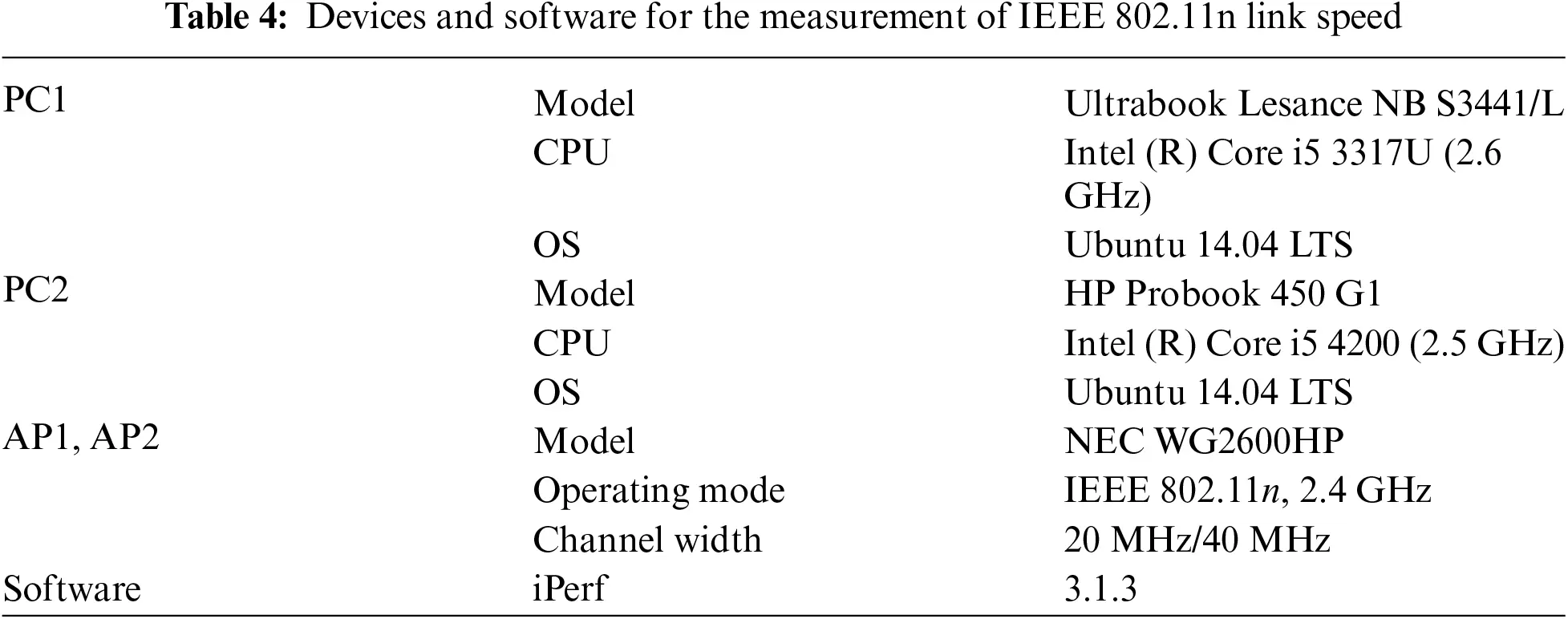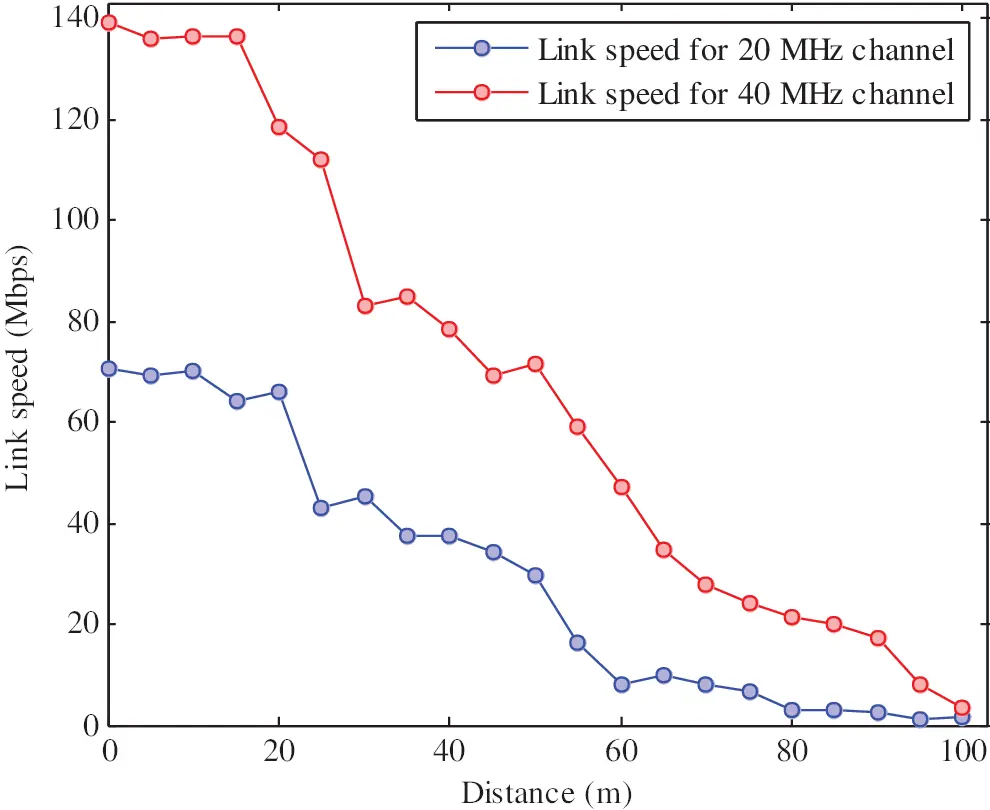Figure 3: IEEE 802.11n link speed measurement results for 20 and 40 MHz channels

Both link speed and RSSI drastically drops sharply with the increase of transmission distance. Such stair-like changes in link speed and RSSI are caused by changes of MCS. The relationship between RSSI and link speed in measurement results is analyzed to yield estimated link speed, spRSSI which is presented as:

where RSSI is the given RSSI and cw is the operating channel width; cw=20 and cw=40 when operating on 20 and 40 MHz channel respectively. The relationship between estimated link speed and RSSI is shown in Figs. 5 and 6 for 20 MHz channel and 40 MHz channel respectively. RSSI of a host from AP can be easily estimated using indoor path loss model [26,27] from transmission distance. The RSSI of host from AP at distance, d (m) is RSSId (dBm) can be derived as:

where RSSI0 presents RSSI at 0m transmission distance between AP and host, α presents path loss exponent, nk presents the number of k-type obstacles on the transmission path and Wk presents the signal attenuation factor (dB) for the k-type obstacle.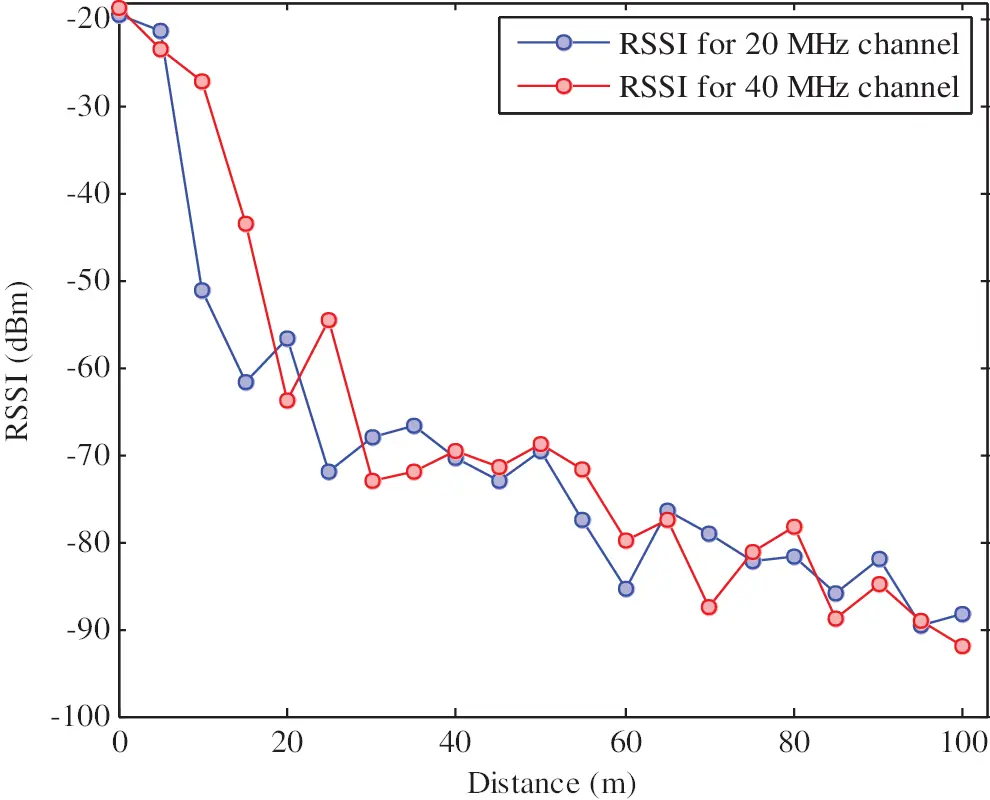Figure 4: IEEE 802.11n RSSI measurement results for 20 and 40 MHz channels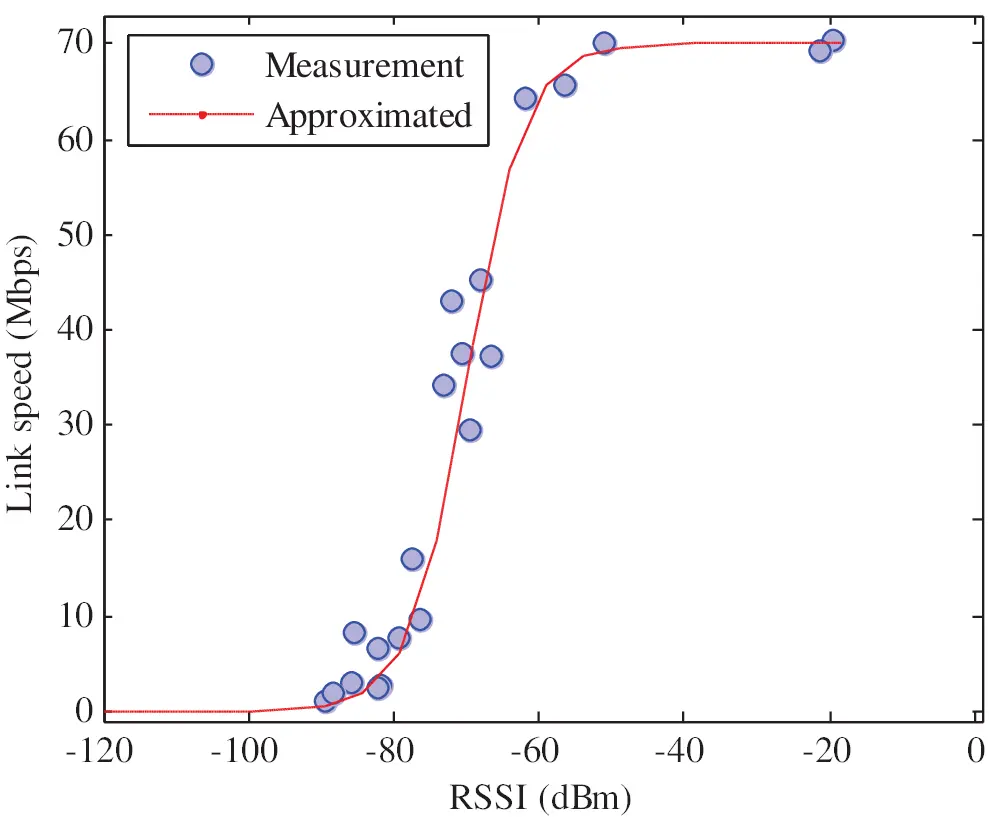Figure 5: Link speed estimation from the measured RSSI for 20 MHz channel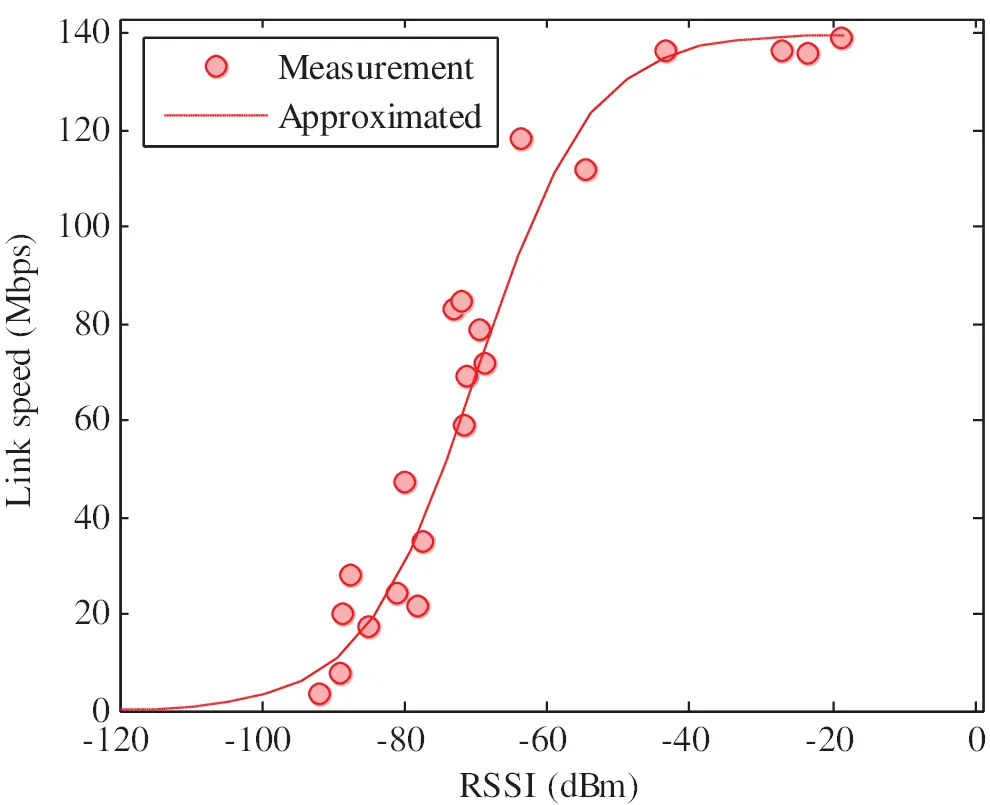Figure 6: Link speed estimation from the measured RSSI for 40 MHz channel

3  Proposed Channel Assignment Algorithm

In this section, we propose channel assignment algorithm to solve hierarchical optimization problem defined in Eq. (7) with an aim to minimize total interference in network. As depicted in Fig. 7, the proposed algorithm comprises four phases namely preprocessing, initial solution generation, iterative improvement and balancing load on channels. The preprocessing phase constructs an interference graph from the set of APs in network. Then, list of interfered APs for each AP is generated and an initial solution is developed using a greedy approach. The solution is iteratively improved using simulated annealing algorithm. Finally, load on each channel is averaged to improve network performance. In the following subsections, we respectively describe each phase of implementation.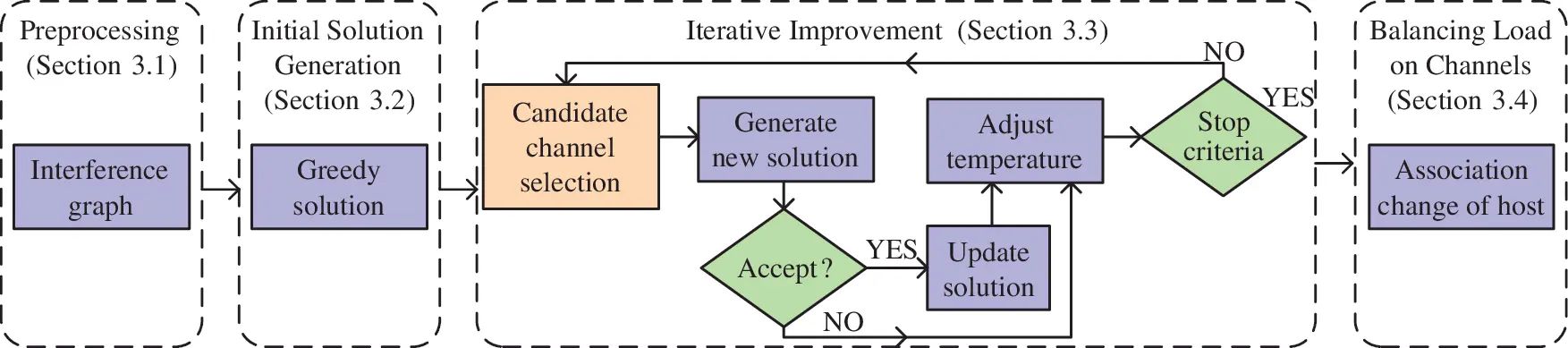Figure 7: Flow chart of proposed channel assignment algorithm

3.1 Channel Assignment Algorithm Step1: Preprocessing

In this section, we model an interference graph from set of APs and interference among them. Let, G=(V,E) be an interference graph where V represents the set of APs and the edge E presents the existence of the interference in any pair of APs in network. An edge, e(i,j)E if APi is interfered with APj. In this work, we consider minimum RSSI of −82 dBm for interference. The weight of an edge presents the degree of inference between two interfered APs e.g., the degree of interference between APi and APj is presented by ρij which can be derived using Eq. (1). After interference graph, necessary information of hierarchical optimization in Eq. (7) is derived for further processing as follows:

Step 1: Preprocessing

① Calculate link speed of any pair AP and host in network. Link speed, spij between APi and hostj can be derived from distance or RSSI using Eqs. (8) and (9).

② Calculate communication time for every AP in network. The communication time τi of APi is defined as the required time of an AP to transmit 1-bit to all its connected hosts and calculated using Eq. (5).

③ Generate list of interfered APs for each AP. The list of interfered APs, L(i) for APi can be derived as:

a) Initialize L(i){i} for APi.

b) For each APjA include j in the list if it is interfered with APi. Therefore, L(i)L(i){j} if e(i,j)=1 and ij.

3.2 Channel Assignment Algorithm Step 2: Initial Solution Generation

In the second step, an initial solution is generated. A straight forward method is to test all possibilities of channel assignment to every AP in the network. For m number of APs and k number of channels, there will be mk number of possibilities. The complexity becomes exponential for such exhaustive method which is not suitable in practical applications for large network. To avoid such exponential cost, we choose a faster greedy method for generation of initial solution. At every step, select a channel for assigning to an AP that minimizes current communication time considering interference. The detail of the procedure is given below:

Step 2: Initial Solution Generation

① Calculate interfered communication time τ^i for each AP using (4) and sort APs in descending order of τ^i. In case of equality, resolve using τi.

② Select a channel cC for APi such that interfered communication time becomes minimum when assigned. Interfered communication time for APi for selected channel is derived by:

τ^iτi+kL(i),kick=cτk×(dmaxdk,i)dmax×ρki where channel ck is allocated to APk.

③ Repeat this greedy selection for channel until each AP is allocated a channel that minimizes current interfered communication time.

④ Calculate total cost Ftot using Eq. (7) which is considered as the initial solution in this work.

3.3 Channel Assignment Algorithm Step 3: Iterative Improvement

In this step, initial solution generated in previous step is improved iteratively using simulated annealing (SA) algorithm. Greedy method for channel selection makes a locally optimal choice aiming to minimize overall interference. However, this does not yield an optimal solution. Therefore, herein, we utilize probabilistic technique of SA to approximate minimal interference. SA starts with the initial solution, selects an AP and a channel with random choice, and examines the changes in total interference. We keep the new selection if it reduces the total interference. Otherwise, we generate a random value between [0,1] and accept or reject the new selection with some probability based on the generated random value, changes in total interference and a given constant namely temperature TSA for the iterative repeating time RTSA. The detail of the iterative step is given below:

Step 3: Iterative Improvement

① Select initial solution in the previous step as the current solution, Ftotold.

② Select one AP and one new channel as an alternative channel assignment. Calculate cost function with new channel, Ftotnew using Eq. (7).

③ Calculate ΔFFtotnewFtotold. If ΔF0, accept the new channel assignment of AP and keep this as the best solution.

④ Otherwise, generate a pseudo-random value, rϵ[0,1] and a probability value, p=eΔFTSA.

a)   IF: r<p , then accept the new channel assignment and Ftotnew as the best solution.

b)   ELSE: keep previous channel assignment and Ftotold as the best solution.

3.4 Channel Assignment Step 4: Balancing Load on Channels

In this step, we aim to balance load on operating channels. After allocation of channels to APs, some APs may become congested and some APs may not. Therefore, balancing of loads on channels is necessary to decrease network traffic and utilize channel capacity more efficiently. For this purpose, it is required to compute network load based on channels. Then, the load of channels can be balanced by changing associations of hosts in intersection of APs to low crowded AP. Thus, network performance achieved in previous step is further improved by applying procedure of averaging load on channels. The detail of the procedure is given below:

Step 4: Balancing Load on Channels

① Select an AP say APi that has the largest interfered communication time, τ^i. A host associated to this AP is considered to change its association to another AP operating on different channel.

② Select a host say hostj associated to APi that satisfies the following conditions:

a)

The host is under coverage of another AP operating on a different channel other than APi.

b)

Link speed, spij from APi to hostj is the smallest among all hosts associated to APi.

③ Select an AP say APk operating different channel than APi for the association of newly selected hostj where link speed spkj is highest among associable APs.

④ Calculate cost function in Eq. (7) considering hostj is associated to APk. If this new association improves network performance, then keep this as the new solution. Otherwise, select another host from Host(i) and repeat from Step ②.

4  Evaluation

In this section, the efficiency of the proposal is verified using WIMNET simulator . First, the accuracy of the throughput estimation is evaluated using proposed interference model. Then, the proposed channel assignment algorithm is evaluated under different network environments by using WIMNET simulator. The simulation is conducted on a platform of Intel (R) Core (TM) i5-4200 CPU @2.5 GHz, 8 GB memory with Ubuntu 14.04 LTS as virtual operating system in VMware workstation. The parameters used in simulation are summarized in Tab. 5. In this work, we simulate only downlink transmission where 104 packets are transmitted from AP to host. We consider two topologies in this work for simulation to represent random and regular network environments respectively. Link speed between any two nodes is calculated using Eq. (8). We assume that the locations of node information are available to derive distance between any pair of nodes in the network. The link speed can also be estimated by using the RSSI as well.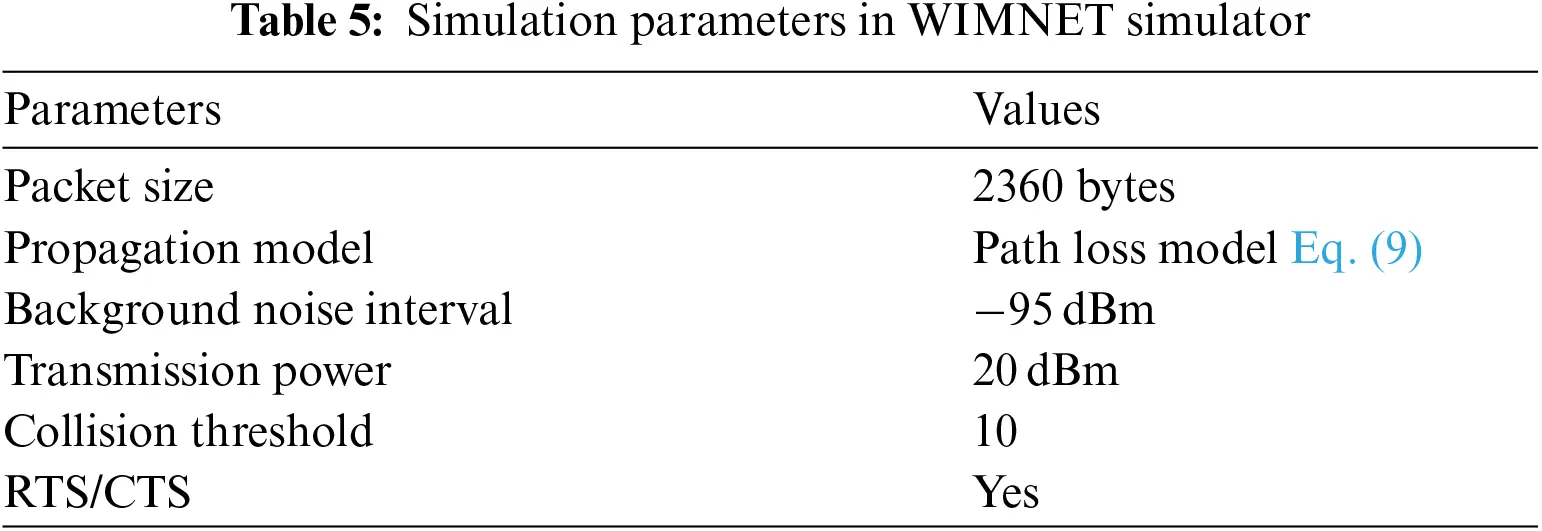4.1 Evaluation of Proposed Interference Model

The proposed interference model is utilized in channel assignment algorithm where link speed is estimated considering interference of neighbor APs using the proposed interference. Therefore, the accuracy of the interference model is needed to verify before using in channel assignment algorithm. To verify the efficiency, we conduct a measurement campaign to collect actual link speed for different channel differences of an AP and its interfered APs at different physical distances. We considered 1 and 50 m physical distances for this work. Then, we compare the estimated link speed using proposed interference model with the actual link speed. The comparison results are shown in Figs. 8 and 9 non-bonded and bonded channels respectively. The estimated link speed using proposed interference model and measured link speed of the same link are shown in y-axis where channel distance ranging from 0 to 8 is shown in x-axis. The results indicate that the estimated results coincide with the measured results. However, the results deviate noticeably at certain places because of signals from unknown APs in of the building. It should be noted that we conducted the measurement experiment at night in weekend when university is closed aiming to minimize the interference from existing Wi-Fi signals.

4.2 Evaluation of Proposed Interference Model

First, we consider a random topology as depicted in Fig. 10 where 12 APs and 60 hosts are distributed in a 250 m × 200 m rectangular area. The APs are distributed equally in the grids of this rectangular network field. We assume that each AP has the same coverage of 100 m. The hosts are generated randomly in the field where a host is associated with an AP that provides maximum RSSI.

Next, we consider a regular network topology, as depicted in Fig. 11. The regular topology models 3rd floor of engineering building-3 in Okayama University, Japan. There are 6 rooms with 2 different room sizes: 7 m× 6 m and 3.5 m × 6 m. We allocated 60 hosts and 9 APs in this dense network environment. The APs and hosts in this topology are regularly distributed since students have their own positions in research rooms. RSSI drops when passing through concrete walls. We consider two types of attenuation factor due to obstacles namely concrete wall and door wall in propagation model Eq. (9). We model such phenomenon by reducing the RSSI 15(dBm) and 3(dBm) if a wall exists between two nodes for concrete wall and door wall respectively.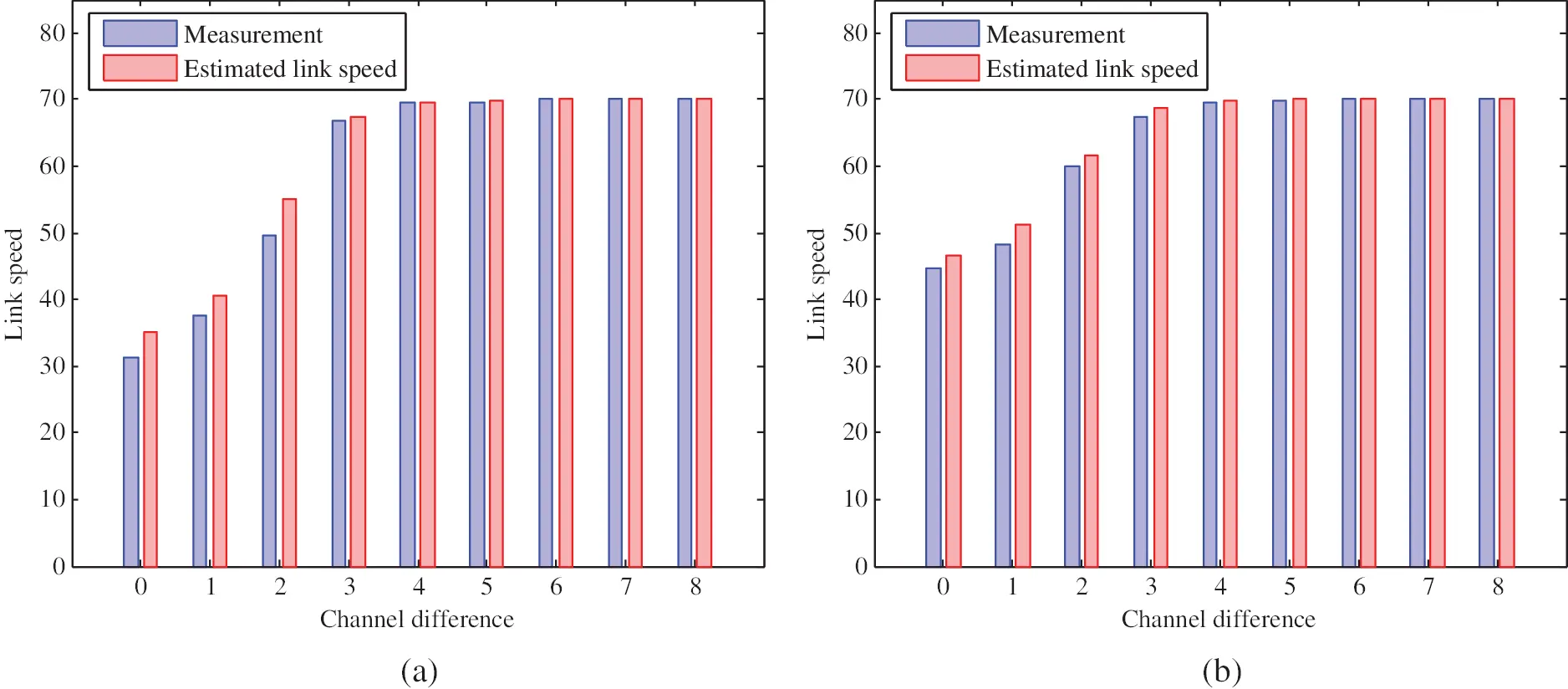Figure 8: Measurement and estimated link speed of 20 MHz channel for different channel difference. (a) AP-AP distance is 1 m (b) AP-AP distance is 50 m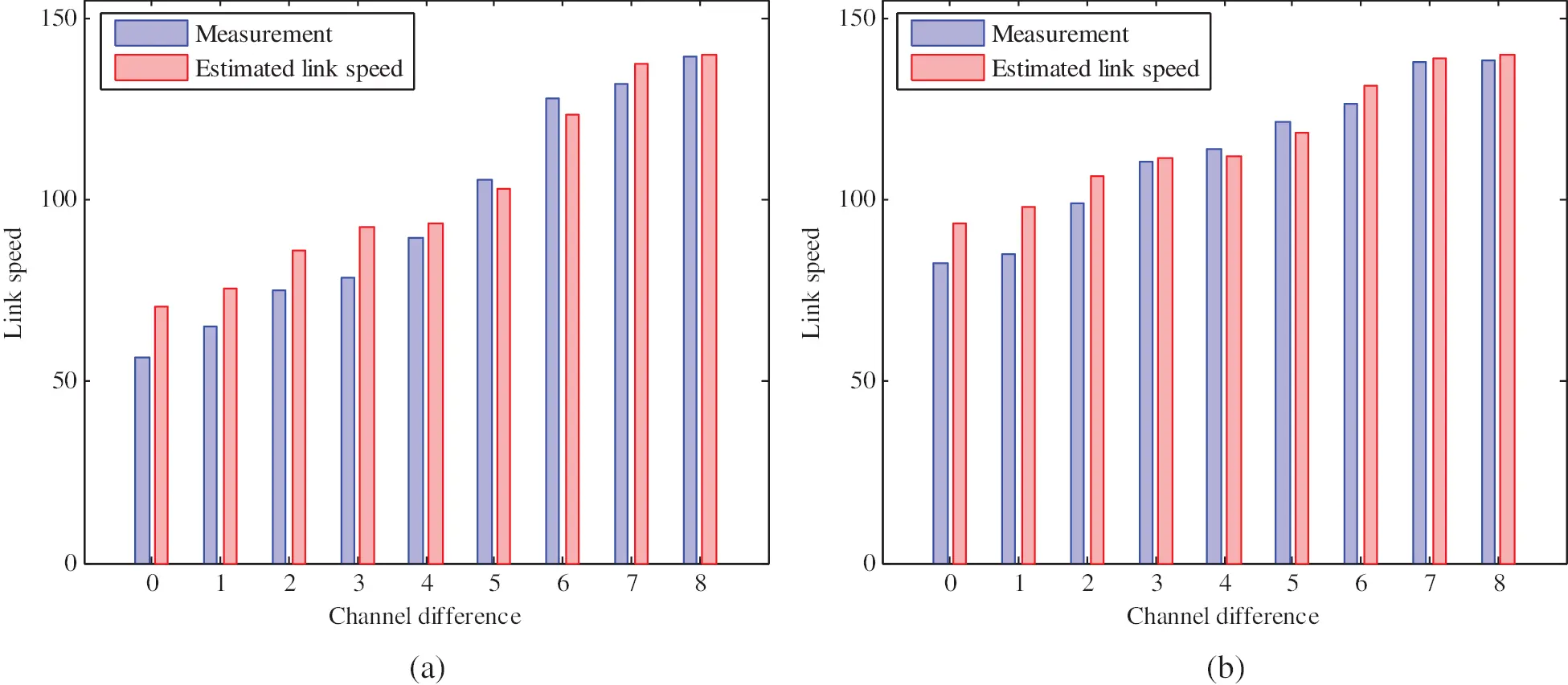Figure 9: Measurement and estimated link speed of 40 MHz channel for different channel difference. (a) AP-AP distance is 1 m (b) AP-AP distance is 50 m

Now, to evaluate proposed channel assignment algorithm, we compare network throughputs obtained by random assignment of channels, greedy channel assignment and proposed algorithm assignment. Here, the random channel assignment experiment is repeated 10 times and average of the network throughputs is considered for evaluation to avoid any kind of biasness. Fig. 12 shows simulation results of network throughput for random and regular topology respectively.Figure 10: Random topology with 12 APs and 60 hosts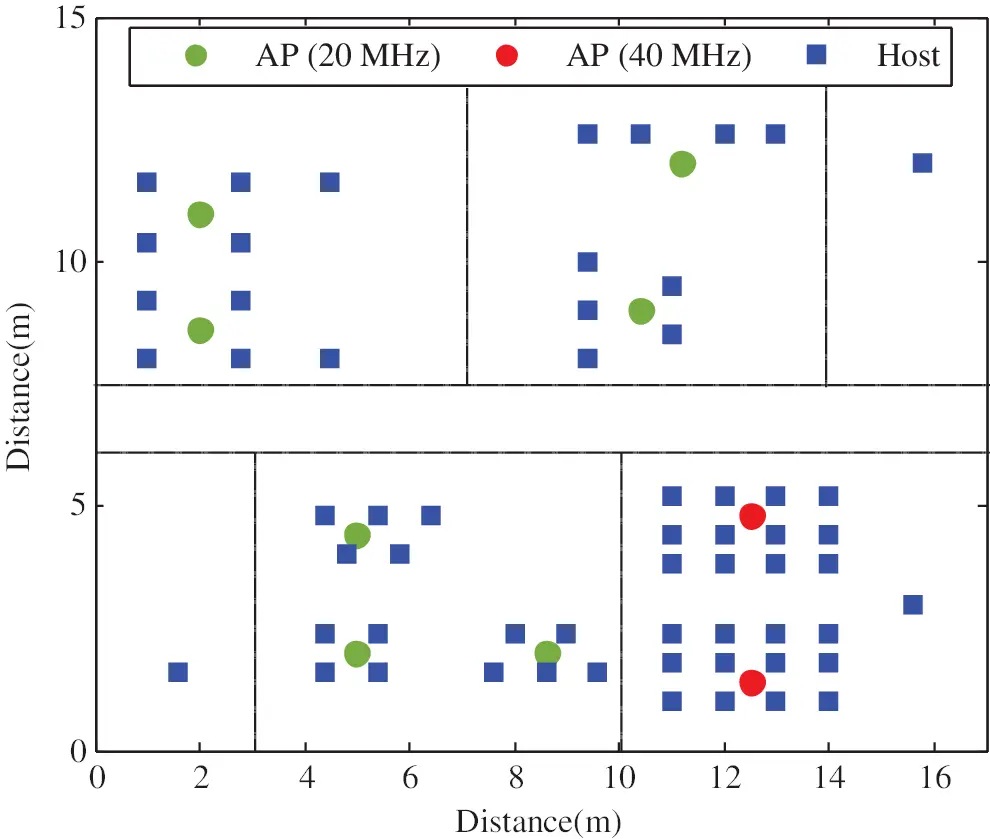Figure 11: Regular topology with 9 APs and 60 hosts

The throughput for combination of bonded and non-bonded channels is expected to be better for flexibility of selection of partial overlapping channels. The numerical results in Fig. 12 demonstrate that the proposed algorithm can successfully assign channels in both topologies to reduce interference compared to random and greedy assignment. The network throughput is comparatively higher in random topology than that of regular topology. This is because, the regular topology is denser than random topology and all the APs are within the coverage of each other. Nonetheless, even in such extreme dense network environment, proposed algorithm works well in assigning channels to improve network performance by reducing overall network interference.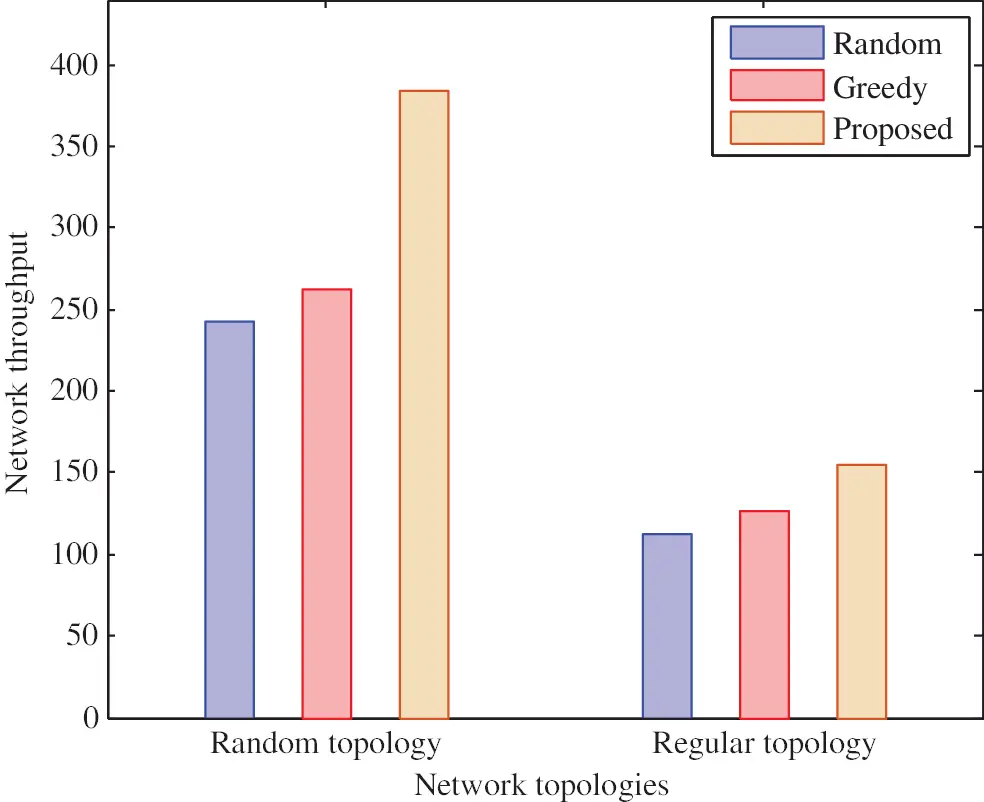Figure 12: Comparison results of random and regular topologies among random, greedy and proposed channel assignment algorithms

5  Conclusion

In this work, we study interference of channels to improve network performance by reducing interference. We propose an interference model for IEEE 802.11n protocol under partially overlapping channels. The proposed model addresses interference of both non-bonded channel and bonded channel. We formulate a hierarchical optimization problem of channel assignment for minimization of total interference in the network. It has been found that reducing the network interference is almost proportional to increasing overall performance of the network. Then, a channel assignment algorithm of partially overlapping channels is introduced using proposed channel interference model in WLAN system. The experimental results showed that the proposed channel assignment algorithm successfully selects the best channel combination and optimizes the network performance. We believe that proposed interference model and algorithm open a new door for installation of WLAN system that specially benefits large-scale network where numerous APs are deployed. The proposal is verified by numerical experiments using WIMNET simulator under several network topologies. In future, we will consider other performance metrics e.g., transmission power, jitter for further improvement of proposed algorithm and dynamic channel width selection in order to improve network performance.

Acknowledgement: Authors would like to thank those who contributed to accomplish this work and provide us with valuable comments.

Funding Statement: The authors received no specific funding for this study.

Conflicts of Interest: The authors declare that they have no conflict of interest to report regarding the present study.

## References

1. S. Manzoor, Z. Chen, Y. Gao, X. Hei and W. Cheng, “Towards QoSaware load balancing for high density software defined Wi-Fi networks,” IEEE Access, vol. 8, pp. 117623–117638, 2020.
2. H. S. Oh, D. G. Jeong and W. S. Jeon, “Joint radio resource management of channel-assignment and user-association for load balancing in dense WLAN environment,” IEEE Access, vol. 8, pp. 69615–69628, 2020.
3. S. Kim, K. Lee, Y. Kim, J. Shin, S. Shin et al., “Dynamic control for on-demand interference-managed WLAN infrastructures,” IEEE/ACM Transactions on Networking, vol. 28, no. 1, pp. 84–97, 2019.
4. R. Karmakar, S. Chattopadhyay and S. Chakraborty, “Novel AP association and fair channel access in high throughput WLAN for energy efficiency,” Ad Hoc Networks, vol. 103, pp. 1–18, 2020.
5. M. S. A. Mamun, N. Funabiki, K. S. Lwin, M. E. Islam and W. C. Kao, “A channel assignment extension of active access-point configuration algorithm for elastic WLAN system and its implementation using raspberry Pi,” International Journal of Networking and Computing, vol. 7, no. 2, pp. 248–270, 2017.
6. C. C. Gómez, I. M. Maestre, J. M. G. Guzman and S. S. Sanz, “A coral reefs optimization algorithm with substrate layer for robust Wi-Fi channel assignment,” Soft Computing, vol. 23, pp. 12621–12640, 2019.
7. A. Ali and F. A. Khan, “Condition and location-aware channel switching scheme for multi-hop multi-band WLANs,” Computer Networks, vol. 168, pp. 1–12, 2020.
8. L. Deek, E. G. Villegas, E. Belding, S. Lee and K. Almeroth, “Intelligent channel bonding in 802.11n WLANs,” IEEE Transactions on Mobile Computing, vol. 13, no. 6, pp. 1242–1255, 2013.
9. A. Faridi, B. Bellalta and A. Checco, “Analysis of dynamic channel bonding in dense networks of WLANs,” IEEE Transactions on Mobile Computing, vol. 16, no. 8, pp. 2118–2131, 2016.
10. K. Zhou, X. Jia, Y. Chang and X. Tang, “Partially overlapping channel assignment for WLANs using SINR interference model,” International Journal of Communication Systems, vol. 27, no. 11, pp. 3082–3095, 2014.
11. J. Lei, J. Jiang and F. Shang, “Channel assignment mechanism for multiple APs cochannel deployment in high density WLANs,” Wireless Communications and Mobile Computing, vol. 2018, pp. 1–11, 2018.
12. D. Gong, M. Zhao and Y. Yang, “Channel assignment in multi-rate 802.11n WLANs,” in Wireless Communications and Networking Conference, Shanghai, China, pp. 392–397, 2013.
13. B. Kaufmann, F. Baccelli, A. Chaintreau, V. Mhatre, K. Papagiannaki et al., “Measurement-based self organization of interfering 802.11 wireless access networks,” in Int. Conf. on Computer Communications, Anchorage, AK, USA, pp. 1451–1459, 2007.
14. C. C. Hsu, Y. A. Liang, J. L. G. Gomez, C. F. Chou and C. J. Lin, “Distributed flexible channel assignment in WLANs,” in Wireless Communications and Networking Conference, Shanghai, China, pp. 493–498, 2013.
15. H. Zhang, H. Ji and W. Ge, “Channel assignment with fairness for multi-AP WLAN based on distributed coordination,” in Wireless Communications and Networking Conference, Cancun, Mexico, pp. 392–397, 2011.
16. D. Xu, Z. Feng, Y. Li and P. Zhang, “Fair channel allocation and power control for uplink and downlink cognitive radio networks,” in IEEE GLOBECOM Workshops, Houston, TX, USA, pp. 591–596, 2011.
17. Y. Wu, Y. Sun, Y. Ji, J. Mao and Y. Liu, “A joint channel allocation and power control scheme for interference mitigation in high density WLANs,” in Int. Conf. on Communication Technology, Guilin, China, pp. 98–103, 2013.
18. I. Dolinska, M. Jakubowski, A. Masiukiewicz, G. Rzkadkowski and K. Piórczynski, “A minimum-spanning-tree-inspired algorithm for channel assignment in 802.11 networks,” International Journal of Electronics and Telecommunications, vol. 62, no. 4, pp. 379–388, 2016.
19. A. Farsi, N. Achir and K. Boussetta, “WLAN planning: Separate and joint optimization of both access point placement and channel assignment,” Annals of Telecommunications, vol. 70, pp. 263–274, 2015.
20. M. Burton, Channel Overlap Calculations for 80211b Networks. White Paper, London, UK: Cirond Networks Inc., 2002.
21. A. Aditya, J. Glenn, S. Srinivasan and S. Peter, “Self-management in chaotic wireless deployments,” in Int. Conf. on Mobile Computing and Networking, New York, USA, pp. 185–199, 2005.
22. T. D. Chiueh, P. Y. Tsai and I. W. Lai, “Baseband Receiver Design for Wireless MIMO-OFDM Communications,” 2nd ed., Singapore: Wiley-IEEE Press, 2012.
23. M. S. A. Mamun, M. E. Islam and N. Funabiki, “An active access-point configuration algorithm for elastic wireless local-area network system using heterogeneous devices,” International Journal of Networking and Computing, vol. 6, no. 2, pp. 395–419, 2016.
24. K. S. Lwin, N. Funabiki, C. Taniguchi, K. K. Zaw, M. S. A. Mamun et al., “A minimax approach for access point setup optimization in IEEE 802.11n wireless networks,” International Journal of Networking and Computing, vol. 7, no. 2, pp. 187–207, 2017.
25. M. Humphrys, “Continuous output-the sigmoid function,” Accessed: Jul. 20, 2021. [Online]. Available: https://www.computing.dcu.ie,/~humphrys/Notes/Neural/sigmoid.html.
26. T. S. Rappaport, Wireless Communications: Principles and Practice. vol. 2, New Jersey: Prentice hall PTR, 1996.
27. D. B. Faria, “Modeling Signal Attenuation in IEEE 802.11 Wireless LANs,” Technical Report, TR-KP06-0118, California‎, USA: Stanford University, 2005.
28. WiFi Channel Scanner, Accessed: Jul. 20, 2021. [Online]. Available: http://www.wifichannelscanner.com/.
29. iPerf-the ultimate speed test tool for TCP, UDP and SCTP,” Accessed: Jul. 20, 2021. [Online]. Available: https://iperf.fr/.
30. N. Funabiki, “Wireless mesh networks,” London, UK: InTechOpen Publisher, 2011. Accessed: Jul. 20, 2021. [Online]. Available: https://www.intechopen.com/books/wireless-mesh-networks.This work is licensed under a Creative Commons Attribution 4.0 International License, which permits unrestricted use, distribution, and reproduction in any medium, provided the original work is properly cited.